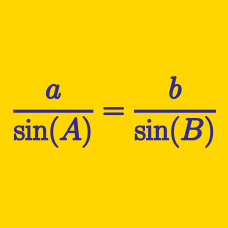Geometry

# Bearing Problems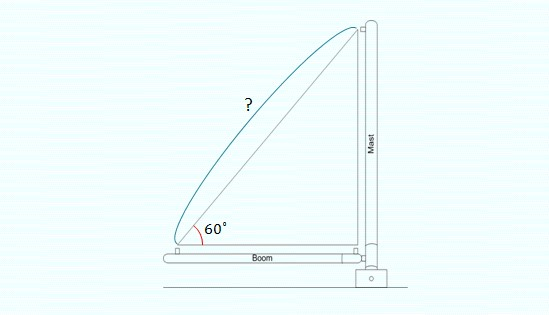The mast in the above diagram is $9$ meters high and a wire is stretched tight to form a straight line to the top of the mast at an angle of $60^\circ.$ How long is the wire in meters?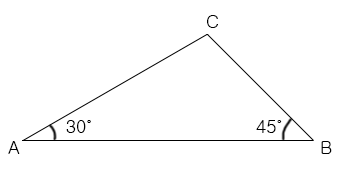The length of $\overline{AC}$ is 4 and the length of $\overline{BC}$ is $2\sqrt{2}$. What is the length of $\overline{AB}$?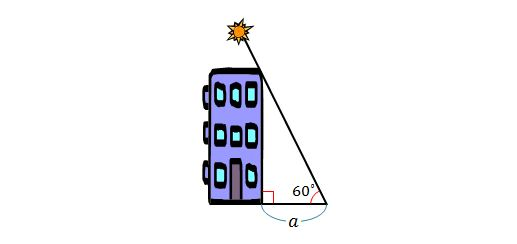In the above diagram, the length of the shadow of the building is $a=32$ meters and the end of the shadow forms an angle of $60^\circ$ with the sun. What is the height of the building?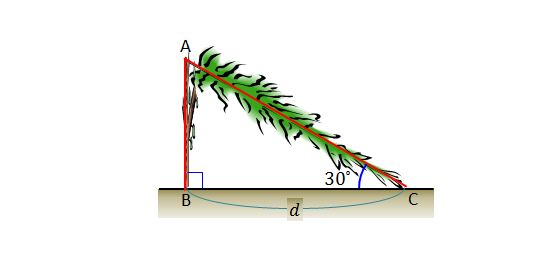A perfectly straight tree is struck by lightning and breaks at point $A$. After falling, the tree forms a triangle with the ground at angle $\angle ACB =30^\circ$ and the distance between the top and bottom of the tree is $d =27\text{ m},$ as illustrated in the above diagram. What is the height of the tree before being struck by lightning?The mast in the above diagram is $21$ meters high and a wire is stretched tight to form a straight line to the top of the mast at an angle of $60^\circ.$ How long is the wire in meters?

×